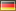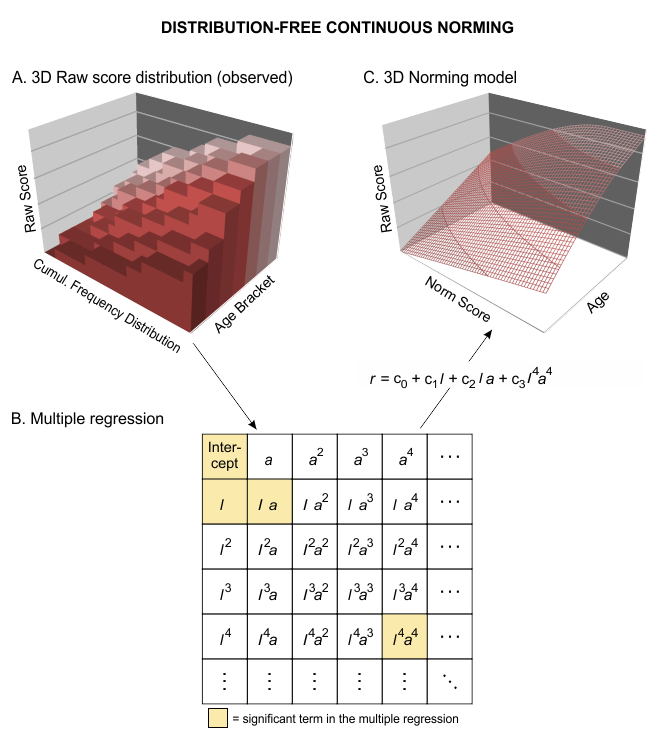## cNORM - Generating Continuous Test Norms

Alexandra Lenhard, Wolfgang Lenhard & Sebastian GarycNORM (W. Lenhard, Lenhard & Gary) is a package for the R environment for statistical computing that aims at generating continuous test norms in psychometrics and biometrics and to analyze the model fit. It is based on the approach of A. Lenhard, Lenhard, Suggate and Segerer (2016).

The method stems from psychometric test construction and was developed to create continuous norms for age or grade in performance assessment (e. g. vocabulary development, A. Lenhard, Lenhard, Segerer & Suggate, 2015; reading and writing development, W. Lenhard, Lenhard & Schneider, 2017). It can however be applied wherever test data like psychological (e. g. intelligence), physiological (e. g. weight) or other measures are dependent on continuous (e.g., age) or discrete (e.g., sex or test mode) explanatory variables. In addition, it can also be used for conventional norm score generation for individual groups, i.e. without modeling depending on other variables.

The package estimates percentile curves in dependence of the explanatory variable (e. g. schooling duration, age ...) via Taylor polynomials, thus offering several advantages:

• By optimizing the model on the basis of the total sample, small deviations from the representativeness of individual subsamples, for example due to incomplete data stratification, are minimized.
• Gaps between different discrete levels of the explanatory variable are closed. For example, in school performance tests, norm tables can be created not only for the discrete measurement point of the normative sample collection (e.g. midyear or end of the year), but also at any time of the school year with the desired accuracy.
• Norm tables are always determined on the basis of the entire normative sample, not only on the basis of a single cohort or class level. Therefore, in comparison to conventional norming, higher norming quality is achieved even with considerably smaller normative samples (W. Lenhard & Lenhard, 2000).
• The limits of the model fit can be evaluated graphically and analytically. For example, it is possible to determine where the model deviates strongly from the manifest data or where strong floor or ceiling effects occur. This makes it possible to specify at which points the test scores can no longer be interpreted in a meaningful way.
• cNORM does not require any distribution assumptions. Therefore, in most use cases the data can be modeled more precisely than with parametric methods (A. Lenhard, Lenhard, & Gary, 2019). This is particularly true for small samples (< 100 per age group or grade) and skewed raw score distributions. Moreover, it applies in particular to those areas that deviate rather strongly from the population average, but often represent precisely those areas that have the highest relevance in diagnostic practice. The computational method can be seen as fitting a hyperplane to the three-dimensional relationship of raw score, norm score and explanatory variable through Taylor polynomials:• In case of violations of represantativeness, Iterative Proportional Fitting (Raking) can be used to calculate weights and apply these to mitigate biases.

For the mathematical background, please have a look at the mathematical derivation of the method.

On the following pages, we demonstrate the necessary steps for the application of the R package with real human performance data, namely, with the standardization sample of the sentence comprehension subtest of ELFE 1-6, a reading comprehension test in German language (W. Lenhard & Schneider, 2006). Essentially, there are five steps to complete:

1. Installation of the R-Package
2. Data Preparation
3. Weighting
4. Data Modeling
5. Model Validation
6. Generating Norm Tables
7. Graphical User Interface Web-based or as a Jamovi Module

### In a nutshell

A quick guide to the essential cNORM functionality:

## Basic example code for modeling the sample dataset

library(cNORM)

# Start the graphical user interface (needs shiny installed)
# The GUI includes the most important functions. For specific cases,
# please use cNORM on the console.

cNORM.GUI()

# If you prefer the console, you can use the syntax as well: Rank data within
# group and compute powers and interactions for the internal dataset 'elfe'
# and compute model. The resulting object includes the ranked data via
# object\$data and model via object\$model.

cnorm.elfe <- cnorm(raw = elfe\$raw, group = elfe\$group)

# Plot R2 of different model solutions in dependence of the number
# of predictors

plot(cnorm.elfe, "subset", type=0) # plot R2
plot(cnorm.elfe, "subset", type=3) # plot MSE

# NOTE! At this point, you usually select a good fitting model and rerun
# the process with a fixed number of terms, e. g. four. Avoid models
# with a high number of terms:

cnorm.elfe <- cnorm(raw = elfe\$raw, group = elfe\$group, terms = 4)

# Usually, age trajectories exhibit a curvilinear shape and thus
# it is sufficient to set the parameter 't' to 3. The power parameter
# for location 'k' can the be increased, e. g. to 5:

cnorm.elfe <- cnorm(raw = elfe\$raw, group = elfe\$group, k = 5, t = 3)

# Visual inspection of the percentile curves of the fitted model

plot(cnorm.elfe, "percentiles")

# Visual inspection of the observed and fitted raw and norm scores

plot(cnorm.elfe, "norm")
plot(cnorm.elfe, "raw")

# In order to check, how other models perform, plot series of percentile
# plots with ascending number of predictors, in this example up to 14
# predictors.

plot(cnorm.elfe, "series", end=14)

# Cross validation of number of terms with 20% of the data for validation
# and 80% training. Due to the time intensity, max terms is restricted to
# 10 in this example; 3 repetitions

cnorm.cv(cnorm.elfe\$data, max=10, repetitions=3)

# Cross validation with pre-specified terms, e. g. of an already
# existing model

cnorm.cv(cnorm.elfe, repetitions=3)

# Print norm table (for grade 3, 3.2, 3.4, 3.6)

normTable(c(3, 3.2, 3.4, 3.6), cnorm.elfe)

# The other way round: Print raw table (for grade 3) together with 90%
# confidence intervalls for a test with a reliability of .94

rawTable(3, cnorm.elfe, CI = .9, reliability = .94)

# cNORM can as well be used for conventional norming. In this case,
# the group variable has to be set to FALSE when ranking the data.
# please use an arbitrary value for age when generating the tables.

d <- rankByGroup(elfe, raw="raw", group=FALSE)
d <- computePowers(d)
m <- bestModel(d)
rawTable(0, model = m)

# start vignette for a complete walk through

vignette("cNORM-Demo", package = "cNORM")

vignette("WeightedRegression", package = "cNORM")

 Installation